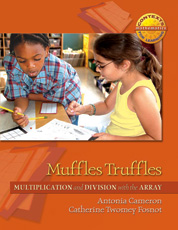# Muffles' Truffles

## Multiplication and Division with the Array

By Antonia Cameron, Catherine Twomey Fosnot

Muffle’s Truffles: Multiplication and Division with the Array is one of five units in the Contexts for Learning Mathematics’ Investigating Multiplication and Division (3–5)

The focus of this unit is the development of the open array as a model for multiplication and division. This unit uses a series of investigations based on the context of Muffles’ Truffles shop. The questions posed in the first investigation (how many boxes of ten can be made with a given quantity of truffles; how many leftovers will there be from a given quantity and how can they
...

### Paperback

In Stock

List Price: \$31.25

Web/School Price: \$25.00

##### PART OF THE SERIES

The Context for Learning Mathematics Series

#### Full Description

Muffle’s Truffles: Multiplication and Division with the Array is one of five units in the Contexts for Learning Mathematics’ Investigating Multiplication and Division (3–5)

The focus of this unit is the development of the open array as a model for multiplication and division. This unit uses a series of investigations based on the context of Muffles’ Truffles shop. The questions posed in the first investigation (how many boxes of ten can be made with a given quantity of truffles; how many leftovers will there be from a given quantity and how can they be combined to make assortment boxes; and what is the cost of a given quantity of truffles if they cost \$1 each) give students an opportunity to explore place value—the multiplicative structure of our base-ten system and quotative division. In the second and third investigations, students build two-dimensional blueprints of one-layer boxes and use these arrays to explore some of the big ideas in multiplication (the distributive, associative, and commutative properties). In the fourth and final investigation, students work with open arrays in the context of labeling and pricing wrapped boxes of truffles. To figure out the dimensions of the wrapped boxes (or open arrays) and the cost, students need to apply a number of big ideas previously developed in this unit.

There are three different kinds of minilessons for multiplication included in the unit as well: counting around the circle, strings of related problems, and quick images. The count-around is used to support the development of place value as it relates to multiplication. The strings of related problems are explicitly designed to guide learners toward computational fluency with whole number multiplication and to build automaticity with multiplication facts by focusing on relationships. The quick images use 2 x 5 and 1 x 5 arrays as units to build larger arrays. In the last days of the unit, more complex minilessons (double-digit multiplication problems) generate a wider range of student strategies that can be explored (and modeled) with the open array.